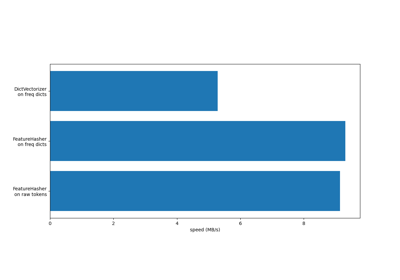# sklearn.feature_extraction.FeatureHasher¶

class sklearn.feature_extraction.FeatureHasher(n_features=1048576, *, input_type='dict', dtype=<class 'numpy.float64'>, alternate_sign=True)[source]

Implements feature hashing, aka the hashing trick.

This class turns sequences of symbolic feature names (strings) into scipy.sparse matrices, using a hash function to compute the matrix column corresponding to a name. The hash function employed is the signed 32-bit version of Murmurhash3.

Feature names of type byte string are used as-is. Unicode strings are converted to UTF-8 first, but no Unicode normalization is done. Feature values must be (finite) numbers.

This class is a low-memory alternative to DictVectorizer and CountVectorizer, intended for large-scale (online) learning and situations where memory is tight, e.g. when running prediction code on embedded devices.

Read more in the User Guide.

New in version 0.13.

Parameters:
n_featuresint, default=2**20

The number of features (columns) in the output matrices. Small numbers of features are likely to cause hash collisions, but large numbers will cause larger coefficient dimensions in linear learners.

input_typestr, default=’dict’

Choose a string from {‘dict’, ‘pair’, ‘string’}. Either “dict” (the default) to accept dictionaries over (feature_name, value); “pair” to accept pairs of (feature_name, value); or “string” to accept single strings. feature_name should be a string, while value should be a number. In the case of “string”, a value of 1 is implied. The feature_name is hashed to find the appropriate column for the feature. The value’s sign might be flipped in the output (but see non_negative, below).

dtypenumpy dtype, default=np.float64

The type of feature values. Passed to scipy.sparse matrix constructors as the dtype argument. Do not set this to bool, np.boolean or any unsigned integer type.

alternate_signbool, default=True

When True, an alternating sign is added to the features as to approximately conserve the inner product in the hashed space even for small n_features. This approach is similar to sparse random projection.

Changed in version 0.19: alternate_sign replaces the now deprecated non_negative parameter.

DictVectorizer

Vectorizes string-valued features using a hash table.

sklearn.preprocessing.OneHotEncoder

Handles nominal/categorical features.

Notes

This estimator is stateless and does not need to be fitted. However, we recommend to call fit_transform instead of transform, as parameter validation is only performed in fit.

Examples

>>> from sklearn.feature_extraction import FeatureHasher
>>> h = FeatureHasher(n_features=10)
>>> D = [{'dog': 1, 'cat':2, 'elephant':4},{'dog': 2, 'run': 5}]
>>> f = h.transform(D)
>>> f.toarray()
array([[ 0.,  0., -4., -1.,  0.,  0.,  0.,  0.,  0.,  2.],
[ 0.,  0.,  0., -2., -5.,  0.,  0.,  0.,  0.,  0.]])


With input_type="string", the input must be an iterable over iterables of strings:

>>> h = FeatureHasher(n_features=8, input_type="string")
>>> raw_X = [["dog", "cat", "snake"], ["snake", "dog"], ["cat", "bird"]]
>>> f = h.transform(raw_X)
>>> f.toarray()
array([[ 0.,  0.,  0., -1.,  0., -1.,  0.,  1.],
[ 0.,  0.,  0., -1.,  0., -1.,  0.,  0.],
[ 0., -1.,  0.,  0.,  0.,  0.,  0.,  1.]])


Methods

 fit([X, y]) Only validates estimator's parameters. fit_transform(X[, y]) Fit to data, then transform it. get_params([deep]) Get parameters for this estimator. set_output(*[, transform]) Set output container. set_params(**params) Set the parameters of this estimator. transform(raw_X) Transform a sequence of instances to a scipy.sparse matrix.
fit(X=None, y=None)[source]

Only validates estimator’s parameters.

This method allows to: (i) validate the estimator’s parameters and (ii) be consistent with the scikit-learn transformer API.

Parameters:
XIgnored

Not used, present here for API consistency by convention.

yIgnored

Not used, present here for API consistency by convention.

Returns:
selfobject

FeatureHasher class instance.

fit_transform(X, y=None, **fit_params)[source]

Fit to data, then transform it.

Fits transformer to X and y with optional parameters fit_params and returns a transformed version of X.

Parameters:
Xarray-like of shape (n_samples, n_features)

Input samples.

yarray-like of shape (n_samples,) or (n_samples, n_outputs), default=None

Target values (None for unsupervised transformations).

**fit_paramsdict

Returns:
X_newndarray array of shape (n_samples, n_features_new)

Transformed array.

get_params(deep=True)[source]

Get parameters for this estimator.

Parameters:
deepbool, default=True

If True, will return the parameters for this estimator and contained subobjects that are estimators.

Returns:
paramsdict

Parameter names mapped to their values.

set_output(*, transform=None)[source]

Set output container.

See Introducing the set_output API for an example on how to use the API.

Parameters:
transform{“default”, “pandas”}, default=None

Configure output of transform and fit_transform.

• "default": Default output format of a transformer

• "pandas": DataFrame output

• None: Transform configuration is unchanged

Returns:
selfestimator instance

Estimator instance.

set_params(**params)[source]

Set the parameters of this estimator.

The method works on simple estimators as well as on nested objects (such as Pipeline). The latter have parameters of the form <component>__<parameter> so that it’s possible to update each component of a nested object.

Parameters:
**paramsdict

Estimator parameters.

Returns:
selfestimator instance

Estimator instance.

transform(raw_X)[source]

Transform a sequence of instances to a scipy.sparse matrix.

Parameters:
raw_Xiterable over iterable over raw features, length = n_samples

Samples. Each sample must be iterable an (e.g., a list or tuple) containing/generating feature names (and optionally values, see the input_type constructor argument) which will be hashed. raw_X need not support the len function, so it can be the result of a generator; n_samples is determined on the fly.

Returns:
Xsparse matrix of shape (n_samples, n_features)

Feature matrix, for use with estimators or further transformers.

## Examples using sklearn.feature_extraction.FeatureHasher¶FeatureHasher and DictVectorizer Comparison

FeatureHasher and DictVectorizer Comparison